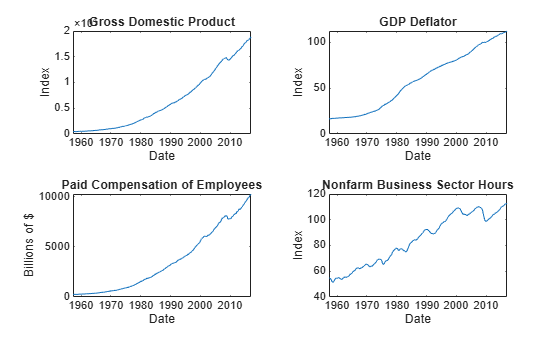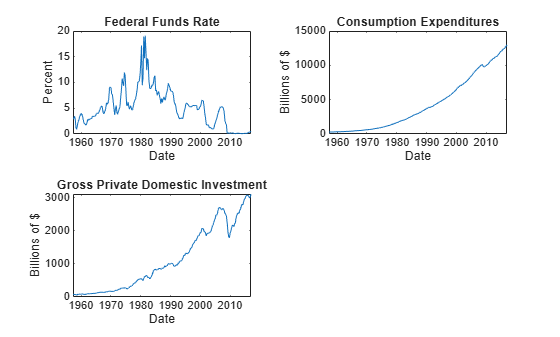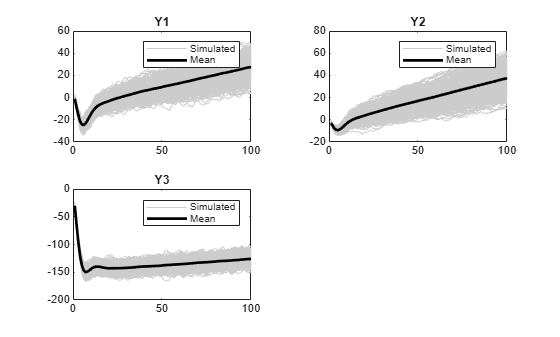simulate

Monte Carlo simulation of vector error-correction (VEC) model

Description

example

Y = simulate(Mdl,numobs) returns a random numobs-period path of multivariate response series (Y) from simulating the fully specified VEC(p – 1) model Mdl.

example

Y = simulate(Mdl,numobs,Name,Value) uses additional options specified by one or more name-value arguments. For example, 'NumPaths',1000,'X',X specifies simulating 1000 paths and X as exogenous predictor data for the regression component.

example

[Y,E] = simulate(___) returns the model innovations E using any of the input arguments in the previous syntaxes.

Examples

collapse all

Consider a VEC model for the following seven macroeconomic series, and then fit the model to the data.

• Gross domestic product (GDP)

• GDP implicit price deflator

• Paid compensation of employees

• Nonfarm business sector hours of all persons

• Effective federal funds rate

• Personal consumption expenditures

• Gross private domestic investment

Suppose that a cointegrating rank of 4 and one short-run term are appropriate, that is, consider a VEC(1) model.

For more information on the data set and variables, enter Description at the command line.

Determine whether the data needs to be preprocessed by plotting the series on separate plots.

figure;
subplot(2,2,1)
plot(FRED.Time,FRED.GDP);
title('Gross Domestic Product');
ylabel('Index');
xlabel('Date');
subplot(2,2,2)
plot(FRED.Time,FRED.GDPDEF);
title('GDP Deflator');
ylabel('Index');
xlabel('Date');
subplot(2,2,3)
plot(FRED.Time,FRED.COE);
title('Paid Compensation of Employees');
ylabel('Billions of \$');
xlabel('Date');
subplot(2,2,4)
plot(FRED.Time,FRED.HOANBS);
ylabel('Index');
xlabel('Date');figure;
subplot(2,2,1)
plot(FRED.Time,FRED.FEDFUNDS);
title('Federal Funds Rate');
ylabel('Percent');
xlabel('Date');
subplot(2,2,2)
plot(FRED.Time,FRED.PCEC);
title('Consumption Expenditures');
ylabel('Billions of \$');
xlabel('Date');
subplot(2,2,3)
plot(FRED.Time,FRED.GPDI);
title('Gross Private Domestic Investment');
ylabel('Billions of \$');
xlabel('Date');Stabilize all series, except the federal funds rate, by applying the log transform. Scale the resulting series by 100 so that all series are on the same scale.

FRED.GDP = 100*log(FRED.GDP);
FRED.GDPDEF = 100*log(FRED.GDPDEF);
FRED.COE = 100*log(FRED.COE);
FRED.HOANBS = 100*log(FRED.HOANBS);
FRED.PCEC = 100*log(FRED.PCEC);
FRED.GPDI = 100*log(FRED.GPDI);

Create a VECM(1) model using the shorthand syntax. Specify the variable names.

Mdl = vecm(7,4,1);
Mdl.SeriesNames = FRED.Properties.VariableNames
Mdl =
vecm with properties:

Description: "7-Dimensional Rank = 4 VEC(1) Model with Linear Time Trend"
SeriesNames: "GDP"  "GDPDEF"  "COE"  ... and 4 more
NumSeries: 7
Rank: 4
P: 2
Constant: [7×1 vector of NaNs]
Cointegration: [7×4 matrix of NaNs]
Impact: [7×7 matrix of NaNs]
CointegrationConstant: [4×1 vector of NaNs]
CointegrationTrend: [4×1 vector of NaNs]
ShortRun: {7×7 matrix of NaNs} at lag 
Trend: [7×1 vector of NaNs]
Beta: [7×0 matrix]
Covariance: [7×7 matrix of NaNs]

Mdl is a vecm model object. All properties containing NaN values correspond to parameters to be estimated given data.

Estimate the model using the entire data set and the default options.

EstMdl = estimate(Mdl,FRED.Variables)
EstMdl =
vecm with properties:

Description: "7-Dimensional Rank = 4 VEC(1) Model"
SeriesNames: "GDP"  "GDPDEF"  "COE"  ... and 4 more
NumSeries: 7
Rank: 4
P: 2
Constant: [14.1329 8.77841 -7.20359 ... and 4 more]'
Cointegration: [7×4 matrix]
Impact: [7×7 matrix]
CointegrationConstant: [-28.6082 109.555 -77.0912 ... and 1 more]'
CointegrationTrend: [4×1 vector of zeros]
ShortRun: {7×7 matrix} at lag 
Trend: [7×1 vector of zeros]
Beta: [7×0 matrix]
Covariance: [7×7 matrix]

EstMdl is an estimated vecm model object. It is fully specified because all parameters have known values. By default, estimate imposes the constraints of the H1 Johansen VEC model form by removing the cointegrating trend and linear trend terms from the model. Parameter exclusion from estimation is equivalent to imposing equality constraints to zero.

Simulate a response series path from the estimated model with length equal to the path in the data.

rng(1); % For reproducibility
numobs = size(FRED,1);
Y = simulate(EstMdl,numobs);

Y is a 240-by-7 matrix of simulated responses. Columns correspond to the variable names in EstMdl.SeriesNames.

Illustrate the relationship between simulate and filter by estimating a 4-D VEC(1) model of the four response series in Johansen's Danish data set. Simulate a single path of responses using the fitted model and the historical data as initial values, and then filter a random set of Gaussian disturbances through the estimated model using the same presample responses.

For details on the variables, enter Description.

Create a default 4-D VEC(1) model. Assume that a cointegrating rank of 1 is appropriate.

Mdl = vecm(4,1,1);
Mdl.SeriesNames = DataTable.Properties.VariableNames
Mdl =
vecm with properties:

Description: "4-Dimensional Rank = 1 VEC(1) Model with Linear Time Trend"
SeriesNames: "M2"  "Y"  "IB"  ... and 1 more
NumSeries: 4
Rank: 1
P: 2
Constant: [4×1 vector of NaNs]
Cointegration: [4×1 matrix of NaNs]
Impact: [4×4 matrix of NaNs]
CointegrationConstant: NaN
CointegrationTrend: NaN
ShortRun: {4×4 matrix of NaNs} at lag 
Trend: [4×1 vector of NaNs]
Beta: [4×0 matrix]
Covariance: [4×4 matrix of NaNs]

Estimate the VEC(1) model using the entire data set. Specify the H1* Johansen model form.

EstMdl = estimate(Mdl,Data,'Model','H1*');

When reproducing the results of simulate and filter, it is important to take these actions.

• Set the same random number seed using rng.

• Specify the same presample response data using the 'Y0' name-value pair argument.

Set the default random seed. Simulate 100 observations by passing the estimated model to simulate. Specify the entire data set as the presample.

rng default;
YSim = simulate(EstMdl,100,'Y0',Data);

YSim is a 100-by-4 matrix of simulated responses. Columns correspond to the columns of the variables in EstMdl.SeriesNames.

Set the default random seed. Simulate 4 series of 100 observations from the standard Gaussian distribution.

rng default;
Z = randn(100,4);

Filter the Gaussian values through the estimated model. Specify the entire data set as the presample.

YFilter = filter(EstMdl,Z,'Y0',Data);

YFilter is a 100-by-4 matrix of simulated responses. Columns correspond to the columns of the variables in EstMdl.SeriesNames. Before filtering the disturbances, filter scales Z by the lower triangular Cholesky factor of the model covariance in EstMdl.Covariance.

Compare the resulting responses between filter and simulate.

(YSim - YFilter)'*(YSim - YFilter)
ans = 4×4

0     0     0     0
0     0     0     0
0     0     0     0
0     0     0     0

The results are identical.

Consider this VEC(1) model for three hypothetical response series.

$\begin{array}{rcl}\Delta {y}_{t}& =& c+A{B}^{\prime }{y}_{t-1}+{\Phi }_{1}\Delta {y}_{t-1}+{\epsilon }_{t}\\ & & \\ & =& \left[\begin{array}{c}-1\\ -3\\ -30\end{array}\right]+\left[\begin{array}{cc}-0.3& 0.3\\ -0.2& 0.1\\ -1& 0\end{array}\right]\left[\begin{array}{ccc}0.1& -0.2& 0.2\\ -0.7& 0.5& 0.2\end{array}\right]{y}_{t-1}+\left[\begin{array}{ccc}0& 0.1& 0.2\\ 0.2& -0.2& 0\\ 0.7& -0.2& 0.3\end{array}\right]\Delta {y}_{t-1}+{\epsilon }_{t}.\end{array}$

The innovations are multivariate Gaussian with a mean of 0 and the covariance matrix

$\Sigma =\left[\begin{array}{ccc}1.3& 0.4& 1.6\\ 0.4& 0.6& 0.7\\ 1.6& 0.7& 5\end{array}\right].$

Create variables for the parameter values.

Adjustment = [-0.3 0.3; -0.2 0.1; -1 0];
Cointegration = [0.1 -0.7; -0.2 0.5; 0.2 0.2];
ShortRun = {[0. 0.1 0.2; 0.2 -0.2 0; 0.7 -0.2 0.3]};
Constant = [-1; -3; -30];
Trend = [0; 0; 0];
Covariance = [1.3 0.4 1.6; 0.4 0.6 0.7; 1.6 0.7 5];

Create a vecm model object representing the VEC(1) model using the appropriate name-value pair arguments.

'Constant',Constant,'ShortRun',ShortRun,'Trend',Trend,...
'Covariance',Covariance);

Mdl is effectively a fully specified vecm model object. That is, the cointegration constant and linear trend are unknown, but are not needed for simulating observations or forecasting given that the overall constant and trend parameters are known.

Simulate 1000 paths of 100 observations. Return the innovations (scaled disturbances).

numpaths = 1000;
numobs = 100;
rng(1); % For reproducibility
[Y,E] = simulate(Mdl,numobs,'NumPaths',numpaths);

Y is a 100-by-3-by-1000 matrix of simulated responses. E is a matrix whose dimensions correspond to the dimensions of Y, but represents the simulated, scaled disturbances. Columns correspond to the response variable names Mdl.SeriesNames.

For each time point, compute the mean vector of the simulated responses among all paths.

MeanSim = mean(Y,3);

MeanSim is a 100-by-7 matrix containing the average of the simulated responses at each time point.

Plot the simulated responses and their averages.

figure;
for j = 1:Mdl.NumSeries
subplot(2,2,j)
plot(squeeze(Y(:,j,:)),'Color',[0.8,0.8,0.8])
title(Mdl.SeriesNames{j});
hold on
plot(MeanSim(:,j));
xlabel('Time index')
hold off
endInput Arguments

collapse all

VEC model, specified as a vecm model object created by vecm or estimate. Mdl must be fully specified.

Number of random observations to generate per output path, specified as a positive integer. The output arguments Y and E have numobs rows.

Data Types: double

Name-Value Arguments

Specify optional comma-separated pairs of Name,Value arguments. Name is the argument name and Value is the corresponding value. Name must appear inside quotes. You can specify several name and value pair arguments in any order as Name1,Value1,...,NameN,ValueN.

Example: 'Y0',Y0,'X',X uses the matrix Y0 as presample responses and the matrix X as predictor data in the regression component.

Number of sample paths to generate, specified as the comma-separated pair consisting of 'NumPaths' and a positive integer. The output arguments Y and E have NumPaths pages.

Example: 'NumPaths',1000

Data Types: double

Presample responses providing initial values for the model, specified as the comma-separated pair consisting of 'Y0' and a numpreobs-by-numseries numeric matrix or a numpreobs-by-numseries-by-numprepaths numeric array.

numpreobs is the number of presample observations. numseries is the number of response series (Mdl.NumSeries). numprepaths is the number of presample response paths.

Rows correspond to presample observations, and the last row contains the latest presample observation. Y0 must have at least Mdl.P rows. If you supply more rows than necessary, simulate uses the latest Mdl.P observations only.

Columns must correspond to the response series names in Mdl.SeriesNames.

Pages correspond to separate, independent paths.

• If Y0 is a matrix, then simulate applies it to simulate each sample path (page). Therefore, all paths in the output argument Y derive from common initial conditions.

• Otherwise, simulate applies Y0(:,:,j) to initialize simulating path j. Y0 must have at least numpaths pages (see NumPaths), and simulate uses only the first numpaths pages.

By default, simulate sets any necessary presample observations.

• For stationary VAR processes without regression components, simulate sets presample observations to the unconditional mean $\mu ={\Phi }^{-1}\left(L\right)c.$

• For nonstationary processes or models that contain a regression component, simulate sets presample observations to zero.

Data Types: double

Predictor data for the regression component in the model, specified as the comma-separated pair consisting of 'X' and a numeric matrix containing numpreds columns.

numpreds is the number of predictor variables (size(Mdl.Beta,2)).

Rows correspond to observations, and the last row contains the latest observation. X must have at least numobs rows. If you supply more rows than necessary, simulate uses only the latest numobs observations. simulate does not use the regression component in the presample period.

Columns correspond to individual predictor variables. All predictor variables are present in the regression component of each response equation.

simulate applies X to each path (page); that is, X represents one path of observed predictors.

By default, simulate excludes the regression component, regardless of its presence in Mdl.

Data Types: double

Future multivariate response series for conditional simulation, specified as the comma-separated pair consisting of 'YF' and a numeric matrix or array containing numseries columns.

Rows correspond to observations in the simulation horizon, and the first row is the earliest observation. Specifically, row j in sample path k (YF(j,:,k)) contains the responses j periods into the future. YF must have at least numobs rows to cover the simulation horizon. If you supply more rows than necessary, simulate uses only the first numobs rows.

Columns must correspond to the response variable names in Mdl.SeriesNames.

Pages correspond to sample paths. Specifically, path k (YF(:,:,k)) captures the state, or knowledge, of the response series as they evolve from the presample past (Y0) into the future.

• If YF is a matrix, then simulate applies YF to each of the numpaths output paths (see NumPaths).

• Otherwise, YF must have at least numpaths pages. If you supply more pages than necessary, simulate uses only the first numpaths pages.

Elements of YF can be numeric scalars or missing values (indicated by NaN values). simulate treats numeric scalars as deterministic future responses that are known in advance, for example, set by policy. simulate simulates responses for corresponding NaN values conditional on the known values.

By default, YF is an array composed of NaN values indicating a complete lack of knowledge of the future state of all simulated responses. Therefore, simulate obtains the output responses Y from a conventional, unconditional Monte Carlo simulation.

For more details, see Algorithms.

Example: Consider simulating one path of a VEC model composed of four response series three periods into the future. Suppose that you have prior knowledge about some of the future values of the responses, and you want to simulate the unknown responses conditional on your knowledge. Specify YF as a matrix containing the values that you know, and use NaN for values you do not know but want to simulate. For example, 'YF',[NaN 2 5 NaN; NaN NaN 0.1 NaN; NaN NaN NaN NaN] specifies that you have no knowledge of the future values of the first and fourth response series; you know the value for period 1 in the second response series, but no other value; and you know the values for periods 1 and 2 in the third response series, but not the value for period 3.

Data Types: double

Note

NaN values in Y0 and X indicate missing values. simulate removes missing values from the data by list-wise deletion. If Y0 is a 3-D array, then simulate performs these steps.

1. Horizontally concatenate pages to form a numpreobs-by-numpaths*numseries matrix.

2. Remove any row that contains at least one NaN from the concatenated data.

In the case of missing observations, the results obtained from multiple paths of Y0 can differ from the results obtained from each path individually.

For conditional simulation (see YF), if X contains any missing values in the latest numobs observations, then simulate throws an error.

Output Arguments

collapse all

Simulated multivariate response series, returned as a numobs-by-numseries numeric matrix or a numobs-by-numseries-by-numpaths numeric array. Y represents the continuation of the presample responses in Y0.

If you specify future responses for conditional simulation using the YF name-value pair argument, then the known values in YF appear in the same positions in Y. However, Y contains simulated values for the missing observations in YF.

Simulated multivariate model innovations series, returned as a numobs-by-numseries numeric matrix or a numobs-by-numseries-by-numpaths numeric array.

If you specify future responses for conditional simulation (see the YF name-value pair argument), then simulate infers the innovations from the known values in YF and places the inferred innovations in the corresponding positions in E. For the missing observations in YF, simulate draws from the Gaussian distribution conditional on any known values, and places the draws in the corresponding positions in E.

Algorithms

• simulate performs conditional simulation using this process for all pages k = 1,...,numpaths and for each time t = 1,...,numobs.

1. simulate infers (or inverse filters) the innovations E(t,:,k) from the known future responses YF(t,:,k). For E(t,:,k), simulate mimics the pattern of NaN values that appears in YF(t,:,k).

2. For the missing elements of E(t,:,k), simulate performs these steps.

1. Draw Z1, the random, standard Gaussian distribution disturbances conditional on the known elements of E(t,:,k).

2. Scale Z1 by the lower triangular Cholesky factor of the conditional covariance matrix. That is, Z2 = L*Z1, where L = chol(C,'lower') and C is the covariance of the conditional Gaussian distribution.

3. Impute Z2 in place of the corresponding missing values in E(t,:,k).

3. For the missing values in YF(t,:,k), simulate filters the corresponding random innovations through the model Mdl.

• simulate uses this process to determine the time origin t0 of models that include linear time trends.

• If you do not specify Y0, then t0 = 0.

• Otherwise, simulate sets t0 to size(Y0,1)Mdl.P. Therefore, the times in the trend component are t = t0 + 1, t0 + 2,..., t0 + numobs. This convention is consistent with the default behavior of model estimation in which estimate removes the first Mdl.P responses, reducing the effective sample size. Although simulate explicitly uses the first Mdl.P presample responses in Y0 to initialize the model, the total number of observations in Y0 (excluding any missing values) determines t0.

 Hamilton, James D. Time Series Analysis. Princeton, NJ: Princeton University Press, 1994.

 Johansen, S. Likelihood-Based Inference in Cointegrated Vector Autoregressive Models. Oxford: Oxford University Press, 1995.

 Juselius, K. The Cointegrated VAR Model. Oxford: Oxford University Press, 2006.

 Lütkepohl, H. New Introduction to Multiple Time Series Analysis. Berlin: Springer, 2005.